## LetsPlayMaths.Com

WELCOME TO THE WORLD OF MATHEMATICS

# Class-8 Trapezium

Introduction to Trapezium

Properties of Trapezium

Isosceles Trapezium

Properties of Isosceles Trapezium

Trapezium MCQ

Trapezium Worksheets

## Introduction to Trapezium

A quadrilateral in which one pair of opposite sides is parallel is known as trapezium.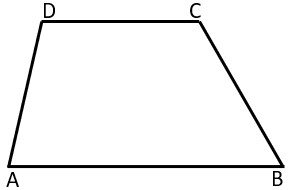In the above figure, ABCD is a quadrilateral in which AB || CD. Hence ABCD is a trapezium. Here AD and BC are not parallel to each other and known as oblique sides

## Properties of Trapezium

1. One pair of opposite sides is parallel to each other
2. Two pair of adjacent angles are supplementary, i.e., ∠A + ∠D = 180° and ∠B + ∠C = 180°

## Isosceles Trapezium

If the two non-parallel sides of a trapezium are equal, then it is known as isosceles trapezium.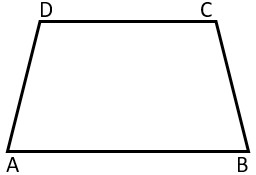## Properties of an Isosceles Trapezium

In an isosceles trapezium ABCD,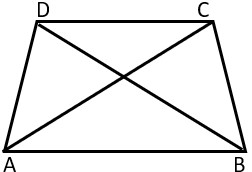1. One pair of opposite sides is parallel i.e., AB || CD.
2. Two pair of adjacent angles are supplementary, i.e., ∠A + ∠D = 180° and ∠B + ∠C = 180°.
3. Angles on the same base are equal, i.e., ∠A = ∠B and ∠C = ∠D.
4. Diagonals are equal in length, i.e., AC = BD.

Example 1. Find the perimeter of the below given isosceles trapezium.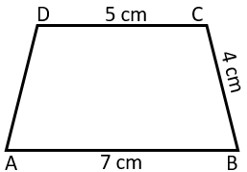Solution. In an isosceles trapezium two non-parallel sides are equal i.e., AD = BC = 4 cm

Perimeter of the ABCD isosceles trapezium = AB + BC + CD + AD

= 7 cm + 4 cm + 5 cm + 4 cm

= 20 cm.

Hence, perimeter of ABCD isosceles trapezium is 20 cm.

Example 2. ABCD is an isosceles trapezium, find the value of ∠A and ∠C.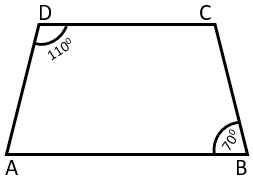Solution. Isosceles trapezium angles on the same base are equal, i.e., ∠A = ∠B = 70° and ∠C = ∠D = 110°.

Hence, ∠A = 70° and ∠C = 110°.

Example 3. ABCD is an isosceles trapezium, find the value of ∠B, ∠C and ∠D.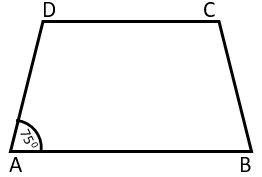Solution. In an isosceles trapezium two pair of adjacent angles are supplementary, i.e., ∠A + ∠D = 180° and ∠B + ∠C = 180°.

∠A + ∠D = 180°

=> 75° + ∠D = 180°

=> ∠D = 180° − 75°

=> ∠D = 105°

As we know isosceles trapezium angles on the same base are equal, i.e., ∠A = ∠B and ∠C = ∠D.

∠A = ∠B = 75°      ∠C = ∠D = 105°

Hence, ∠B = 75°

Example 4. Find the value of p in the below given trapezium ABCD.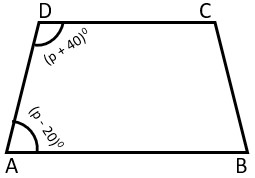Solution. In the given ABCD trapezium ∠A = (p − 20)° and ∠D = (p + 40)°.

As we know, in a trapezium, the angles on either sides of the base are supplementary.

∠A + ∠D = 180°

=> (p − 20) + (p + 40) = 180

=> 2p + 20 = 180

=> 2p = 180 − 20

=> 2p = 160

=> p = 1602

=> p = 80

Hence, the value of p is equal to 80.

Example 5. ABCD is a trapezium such that AB || CD, ∠A : ∠D = 2 : 1 and ∠B : ∠C = 7 : 5. Find the measure of all the angles of the trapezium.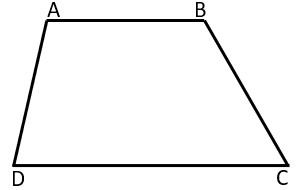Solution. Let's assume ∠A = 2p and ∠D = p.

As we know ∠A and ∠D are supplementary.

∠A + ∠D = 180°

=> 2p + p = 180°

=> 3p = 180°

=> p = 180°3

=> p = 60°

∠A = 2p = 2 × 60° = 120°

∠D = 60°

Let's assume ∠B = 7q and ∠C = 5q are supplementary.

Similarly, ∠B and ∠C are supplementary.

∠B + ∠C = 180°

=> 7q + 5q = 180°

=> 12q = 180°

=> q = 180°12

=> q = 15°

∠B = 7q = 7 × 15° = 105°

∠C = 5q = 5 × 15° = 75°

Hence, ∠A = 120°, ∠B = 105°, ∠C = 75° and ∠D = 60°.

Example 6. In trapezium ABCD, DO and CO are bisectors of ∠D and ∠C respectively. Find ∠A and ∠B.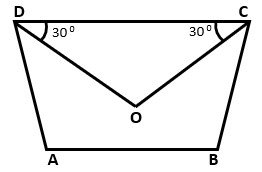Solution. ∠ODC = ∠ODA = 30° and ∠OCD = ∠OCB = 30°

So, ∠D = 60° and ∠C = 60°.

Since, ABCD is a trapezium,

∠A + ∠D = 180° and ∠B + ∠C = 180°

=> ∠A + 60° = 180°    => ∠B + 60° = 180°

=> ∠A = 180° − 60°    => ∠B = 180° − 60°

=> ∠A = 120°    => ∠B = 120°

Hence, ∠A = 120° and ∠B = 120°.

## Class-8 Trapezium MCQ

Trapezium Test - 1

Trapezium Test - 2

## Class-8 Trapezium Worksheets

Trapezium Worksheet - 1

Trapezium Worksheet - 2